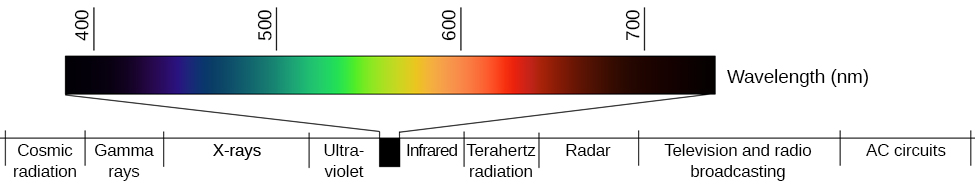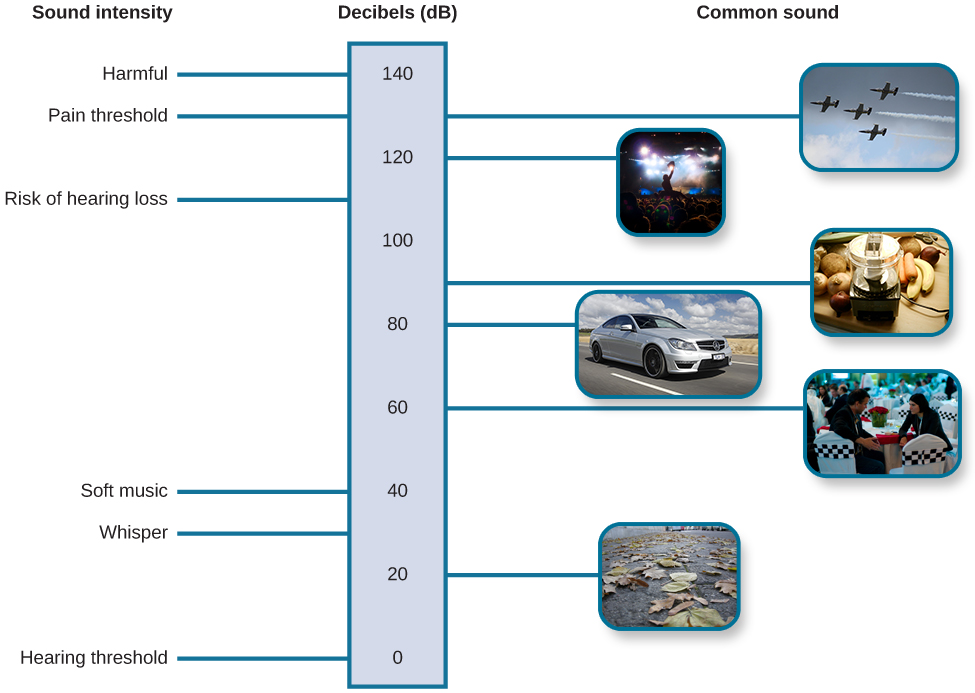# 5.3: Waves and Wavelengths

•• OpenStax
• OpenStax
$$\newcommand{\vecs}{\overset { \rightharpoonup} {\mathbf{#1}} }$$ $$\newcommand{\vecd}{\overset{-\!-\!\rightharpoonup}{\vphantom{a}\smash {#1}}}$$$$\newcommand{\id}{\mathrm{id}}$$ $$\newcommand{\Span}{\mathrm{span}}$$ $$\newcommand{\kernel}{\mathrm{null}\,}$$ $$\newcommand{\range}{\mathrm{range}\,}$$ $$\newcommand{\RealPart}{\mathrm{Re}}$$ $$\newcommand{\ImaginaryPart}{\mathrm{Im}}$$ $$\newcommand{\Argument}{\mathrm{Arg}}$$ $$\newcommand{\norm}{\| #1 \|}$$ $$\newcommand{\inner}{\langle #1, #2 \rangle}$$ $$\newcommand{\Span}{\mathrm{span}}$$ $$\newcommand{\id}{\mathrm{id}}$$ $$\newcommand{\Span}{\mathrm{span}}$$ $$\newcommand{\kernel}{\mathrm{null}\,}$$ $$\newcommand{\range}{\mathrm{range}\,}$$ $$\newcommand{\RealPart}{\mathrm{Re}}$$ $$\newcommand{\ImaginaryPart}{\mathrm{Im}}$$ $$\newcommand{\Argument}{\mathrm{Arg}}$$ $$\newcommand{\norm}{\| #1 \|}$$ $$\newcommand{\inner}{\langle #1, #2 \rangle}$$ $$\newcommand{\Span}{\mathrm{span}}$$

Learning Objectives

• Describe important physical features of wave forms
• Show how physical properties of light waves are associated with perceptual experience
• Show how physical properties of sound waves are associated with perceptual experience

Visual and auditory stimuli both occur in the form of waves. Although the two stimuli are very different in terms of composition, wave forms share similar characteristics that are especially important to our visual and auditory perceptions. In this section, we describe the physical properties of the waves as well as the perceptual experiences associated with them.

## Amplitude and Wavelength

Two physical characteristics of a wave are amplitude and wavelength. The amplitude of a wave is the height of a wave as measured from the highest point on the wave (peak or crest) to the lowest point on the wave (trough). Wavelength refers to the length of a wave from one peak to the next.Figure $$\PageIndex{1}$$: The amplitude or height of a wave is measured from the peak to the trough. The wavelength is measured from peak to peak.

Wavelength is directly related to the frequency of a given wave form. Frequency refers to the number of waves that pass a given point in a given time period and is often expressed in terms of hertz (Hz), or cycles per second. Longer wavelengths will have lower frequencies, and shorter wavelengths will have higher frequencies.Figure $$\PageIndex{2}$$: This figure illustrates waves of differing wavelengths/frequencies. At the top of the figure, the red wave has a long wavelength/short frequency. Moving from top to bottom, the wavelengths decrease and frequencies increase.

## Light Waves

The visible spectrum is the portion of the larger electromagnetic spectrum that we can see. As the figure below shows, the electromagnetic spectrum encompasses all of the electromagnetic radiation that occurs in our environment and includes gamma rays, $$x$$-rays, ultraviolet light, visible light, infrared light, microwaves, and radio waves. The visible spectrum in humans is associated with wavelengths that range from $$380$$ to $$740$$ nm—a very small distance, since a nanometer (nm) is one billionth of a meter. Other species can detect other portions of the electromagnetic spectrum. For instance, honeybees can see light in the ultraviolet range (Wakakuwa, Stavenga, & Arikawa, 2007), and some snakes can detect infrared radiation in addition to more traditional visual light cues (Chen, Deng, Brauth, Ding, & Tang, 2012; Hartline, Kass, & Loop, 1978).Figure $$\PageIndex{3}$$: Light that is visible to humans makes up only a small portion of the electromagnetic spectrum.

In humans, light wavelength is associated with perception of color. Within the visible spectrum, our experience of red is associated with longer wavelengths, greens are intermediate, and blues and violets are shorter in wavelength. (An easy way to remember this is the mnemonic ROYGBIV: red, orange, yellow, green, blue, indigo, violet.) The amplitude of light waves is associated with our experience of brightness or intensity of color, with larger amplitudes appearing brighter.Figure $$\PageIndex{4}$$: Different wavelengths of light are associated with our perception of different colors. (credit: modification of work by Johannes Ahlmann)

## Sound Waves

Like light waves, the physical properties of sound waves are associated with various aspects of our perception of sound. The frequency of a sound wave is associated with our perception of that sound’s pitch. High-frequency sound waves are perceived as high-pitched sounds, while low-frequency sound waves are perceived as low-pitched sounds. The audible range of sound frequencies is between $$20$$ and $$20000$$ Hz, with greatest sensitivity to those frequencies that fall in the middle of this range.

As was the case with the visible spectrum, other species show differences in their audible ranges. For instance, chickens have a very limited audible range, from $$125$$ to $$2000$$ Hz. Mice have an audible range from $$1000$$ to $$91000$$ Hz, and the beluga whale’s audible range is from $$1000$$ to $$123000$$ Hz. Our pet dogs and cats have audible ranges of about $$70-45000$$ Hz and $$45-64000$$ Hz, respectively (Strain, 2003).

The loudness of a given sound is closely associated with the amplitude of the sound wave. Higher amplitudes are associated with louder sounds. Loudness is measured in terms of decibels (dB), a logarithmic unit of sound intensity. A typical conversation would correlate with $$60$$ dB; a rock concert might check in at $$120$$ dB. A whisper $$5$$ feet away or rustling leaves are at the low end of our hearing range; sounds like a window air conditioner, a normal conversation, and even heavy traffic or a vacuum cleaner are within a tolerable range. However, there is the potential for hearing damage from about $$80$$ dB to $$130$$ dB: These are sounds of a food processor, power lawnmower, heavy truck ($$25$$ feet away), subway train ($$20$$ feet away), live rock music, and a jackhammer. The threshold for pain is about $$130$$ dB, a jet plane taking off or a revolver firing at close range (Dunkle, 1982).Figure $$\PageIndex{5}$$: This figure illustrates the loudness of common sounds. (credit "planes": modification of work by Max Pfandl; credit "crowd": modification of work by Christian Holmér; credit "blender": modification of work by Jo Brodie; credit "car": modification of work by NRMA New Cars/Flickr; credit "talking": modification of work by Joi Ito; credit "leaves": modification of work by Aurelijus Valeiša)

Although wave amplitude is generally associated with loudness, there is some interaction between frequency and amplitude in our perception of loudness within the audible range. For example, a $$10$$ Hz sound wave is inaudible no matter the amplitude of the wave. A $$1000$$ Hz sound wave, on the other hand, would vary dramatically in terms of perceived loudness as the amplitude of the wave increased.

Of course, different musical instruments can play the same musical note at the same level of loudness, yet they still sound quite different. This is known as the timbre of a sound. Timbre refers to a sound’s purity, and it is affected by the complex interplay of frequency, amplitude, and timing of sound waves.

## Summary

Both light and sound can be described in terms of wave forms with physical characteristics like amplitude, wavelength, and timbre. Wavelength and frequency are inversely related so that longer waves have lower frequencies, and shorter waves have higher frequencies. In the visual system, a light wave’s wavelength is generally associated with color, and its amplitude is associated with brightness. In the auditory system, a sound’s frequency is associated with pitch, and its amplitude is associated with loudness.

## Glossary

amplitude
height of a wave
decibel (dB)
logarithmic unit of sound intensity
electromagnetic spectrum
all the electromagnetic radiation that occurs in our environment
frequency
number of waves that pass a given point in a given time period
hertz (Hz)
cycles per second; measure of frequency
peak
(also, crest) highest point of a wave
pitch
perception of a sound’s frequency
timbre
sound’s purity
trough
lowest point of a wave
visible spectrum
portion of the electromagnetic spectrum that we can see
wavelength
length of a wave from one peak to the next peak